Application Notes

# Moku:Lab and MATLAB — Using the Arbitrary Waveform Generator with MATLAB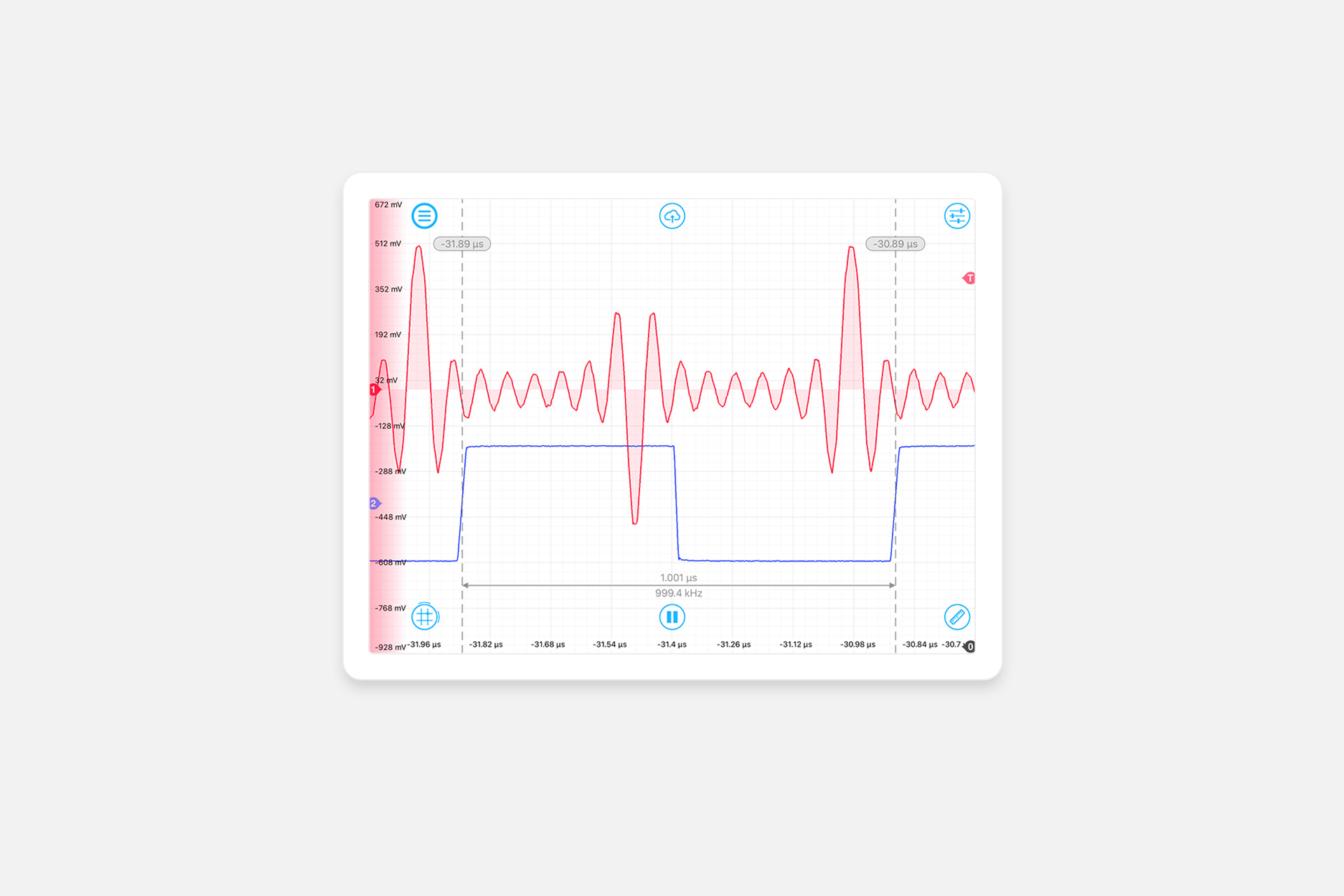In this application note we provide a tutorial using Moku:Lab’s Arbitrary Waveform Generator with MATLAB. Step-by-step instructions are detailed for deploying two simple waveforms from MATLAB directly to Moku:Lab. We use a second Moku:Lab running the Oscilloscope and Spectrum Analyzer instruments to confirm the output waveforms.

A Lissajous curve example is then shown together with a more complex, 2 channel waveform.

MATLAB files to accompany this note are available to download from Liquid Instruments.

## Arbitrary Waveform Generator

Moku:Lab’s Arbitrary Waveform Generator (AWG) can generate custom waveforms with up to 65,536 points at sampling rates of up to 1 GSa/s. Waveforms can be loaded from a file, or input as a piece-wise mathematical function with up to 32 segments, enabling you to generate truly arbitrary waveforms. In this note we will generate the waveform and download it directly to Moku:Lab, entirely within MATLAB.

### Prerequisites and setup

This application note and tutorial assumes you have installed MATLAB and the associated Moku:Lab MATLAB toolbox :

Moku:Lab supports the following MATLAB versions:

• Windows: MATLAB R2013a and later
• Linux: MATLAB R2013a and later
• MacOS: MATLAB 2014a and later

For the toolbox:

MATLAB 2015+

3. Select “Install”
4. Confirm installation with help moku in the MATLAB terminal

MATLAB 2013-2014

2. Extract the zip, typically to `../MATLAB/Add-Ons/Toolboxes`
3. Open MATALB and, in the Home tool pane, click “set Path”
4. Click “Add with Subfolders” and select the folder of the extracted ZIP
5. Click “Save” to add the toolbox folder to the MATLAB path

In this note, we use MATLAB R2019b on MacOS.

### Moku:Lab on MATLAB

In order for MATLAB to control Moku:Lab, the computer running MATLAB must be on the same sub-net/LAN as Moku:Lab. MATLAB needs the IP address of Moku:Lab; you can determine this in one of two ways :

• A Bonjour/zeroconf service discovery utility
• On launch screen of Moku:Lab’s iPad app; push and hold the icon of the Moku:Lab to reveal its IP address

Within MATLAB you can access Moku:Lab documentation with command help moku

The MATLAB toolbox includes numerous example scripts, and these are typically located in the toolbox folder. On MacOS this is located at:

`~/Library/ApplicationSupport/MathWorks/MATLAB Add-Ons/Toolboxes/moku-MaTLAB-2.8.1/examples`

On Windows:

`\Users\...\AppData\Roaming\MathWorks\MATLAB Add-Ons\Toolboxes\moku-MATLAB-2.8.1\examples`

The MATLAB scripts for each of these examples are available for download here:

## Example #1 : Square wave and sine wave with harmonics

For our first example we will deploy the Toolbox example:

`arbitrarywavegen_basic.m`

Referring to this script, we see that the array `square_wave` is defined to be an array of length 100 consisting of values of +1 or -1.

The `not_square_wave` is defined to be an array of length 100 of values describing a sine wave with the addition of several odd-numbered harmonics.

After running the MATLAB script, the user enters an IP address and within the script, the line :

`m = MokuArbitraryWaveGen(ip)`

sets up and deploys an instance of an Arbitrary Waveform Generator instrument on Moku:Lab. Then one call for each channel to

`m.write_lut`

and

`m.gen_waveformfunction`

configures the waveform on the Moku:Lab hardware.

We can view and confirm these waveforms with the MATLAB plotting and graphing commands. Figure 1 shows the square wave in blue and the sine wave plus harmonics in red.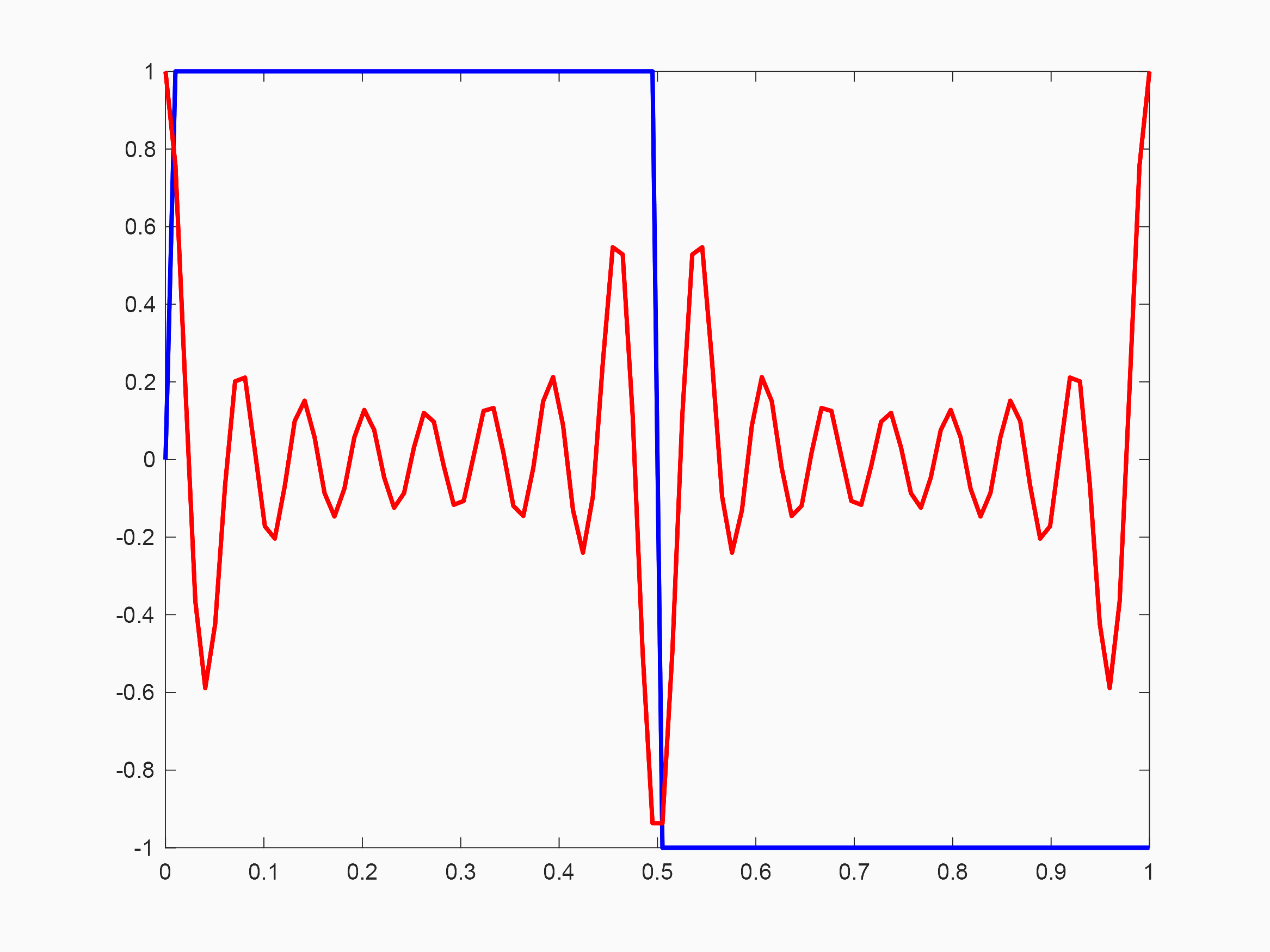Figure 1: MATLAB plot of example waveforms

But, it is more useful to view these waveforms as generated on Moku:Lab. On our lab bench, the top, black Moku:Lab is connected to MATLAB and generating our two signals with the deployed AWG.

The lower blue Moku:Lab is interfaced with an iPad, which is running the Oscilloscope instrument. This setup is shown in Figure 2.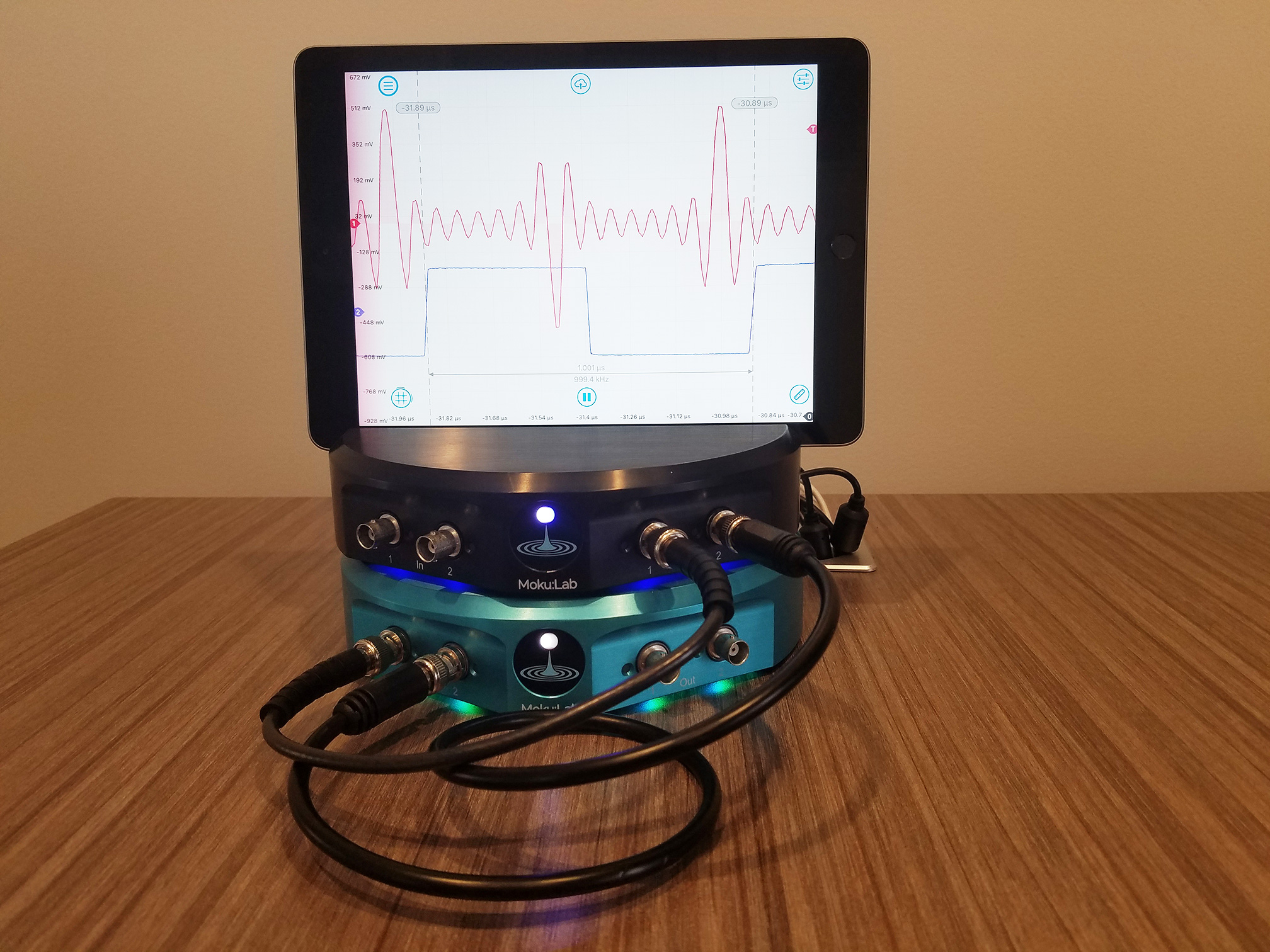Figure 2: Moku:Lab setup

The AWG output channels are connected to the Oscilloscope inputs and we can observe the waveforms on the iPad. The cursors show the expected frequency of 1 MHz (actual measurement on screen of 999.4 kHz). Moku:Lab’s integration with Dropbox allows us to quickly share the screenshot, see Figure 3 (showing the actual AWG output), a square wave on channel 2 (blue), and a sine wave plus harmonics on channel 1 (red).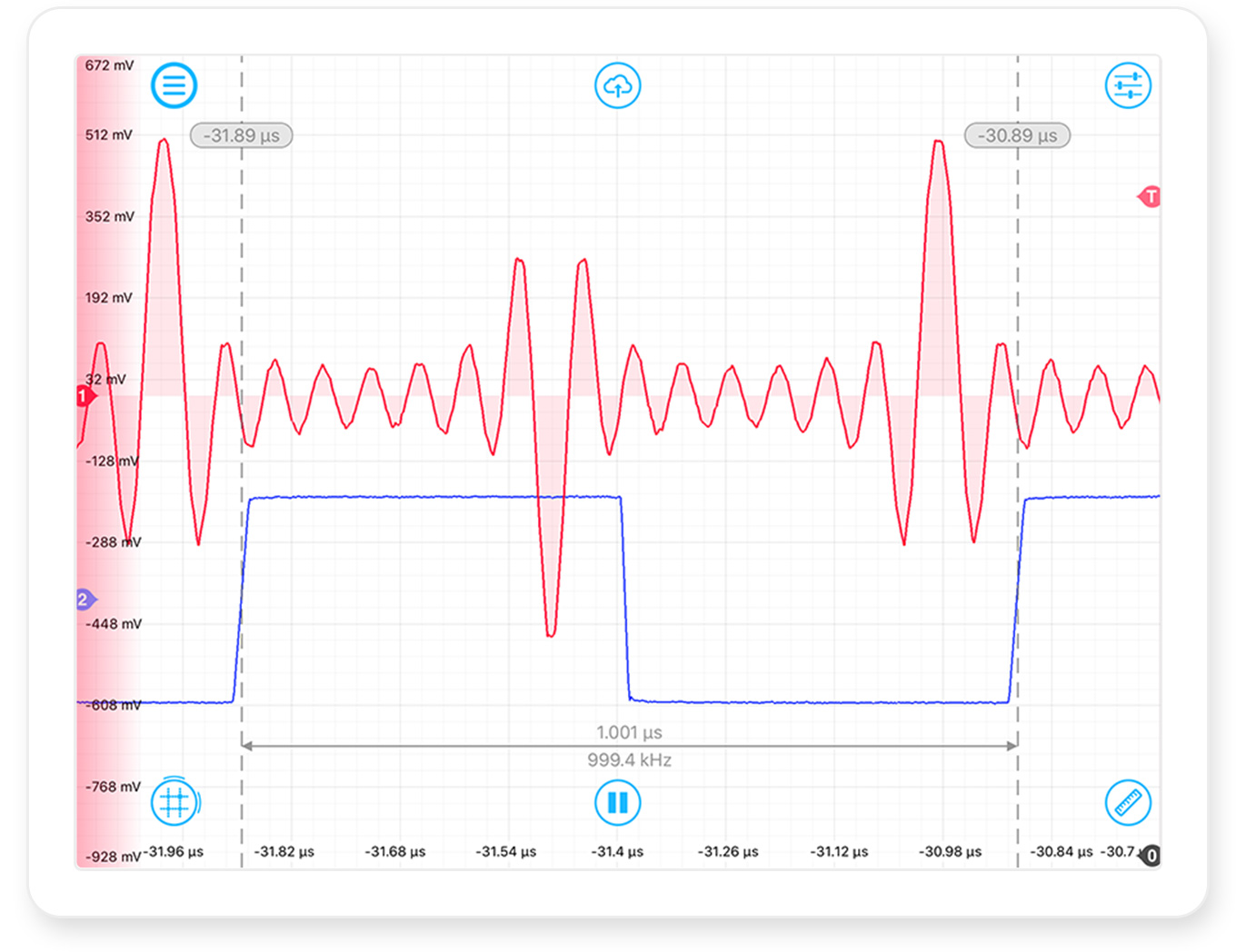Figure 3: Moku:Lab Oscilloscope screenshot, shows the MokuLab AWG as generated from MATLAB

Due to Moku:Lab’s flexibility we can quickly switch the blue Moku:Lab from an Oscilloscope to a Spectrum Analyzer.

The spectrum of the square wave shown in Figure 4 and as expected for a square wave, shows fundamental at 1 MHz and a series of odd harmonics extending to the limits of the plot at 100 MHz.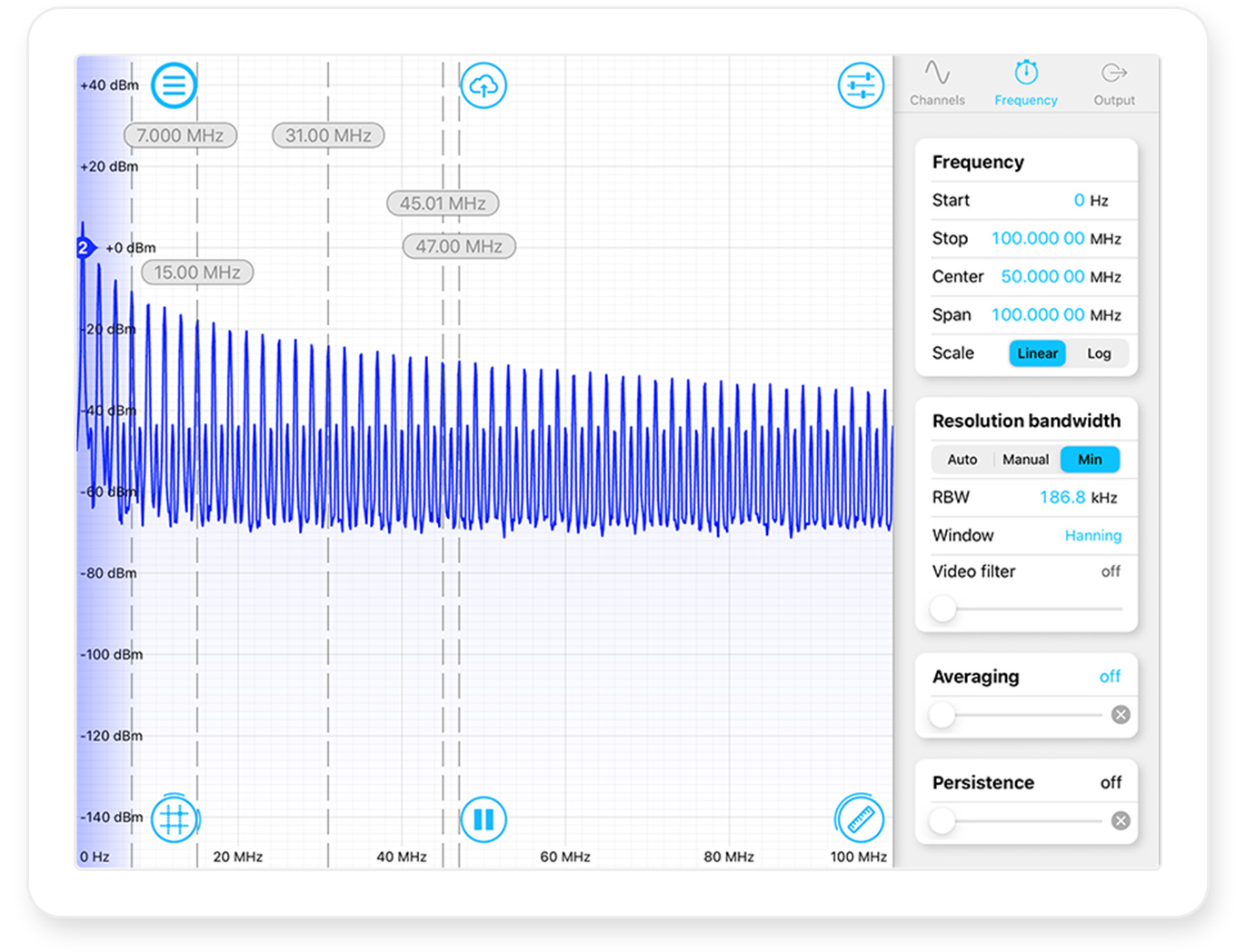Figure 4: Square wave spectrum on Moku:Lab’s Spectrum Analyzer

Our MATLAB script for the not_square_wave essentially sums a sine wave with 7 odd harmonics. The spectrum of not_square_wave is shown in Figure 5 and this shows the 1 MHz fundamental and 7 harmonic peaks extending to 15 MHz. This is labelled on screen with a cursor from the iPad app.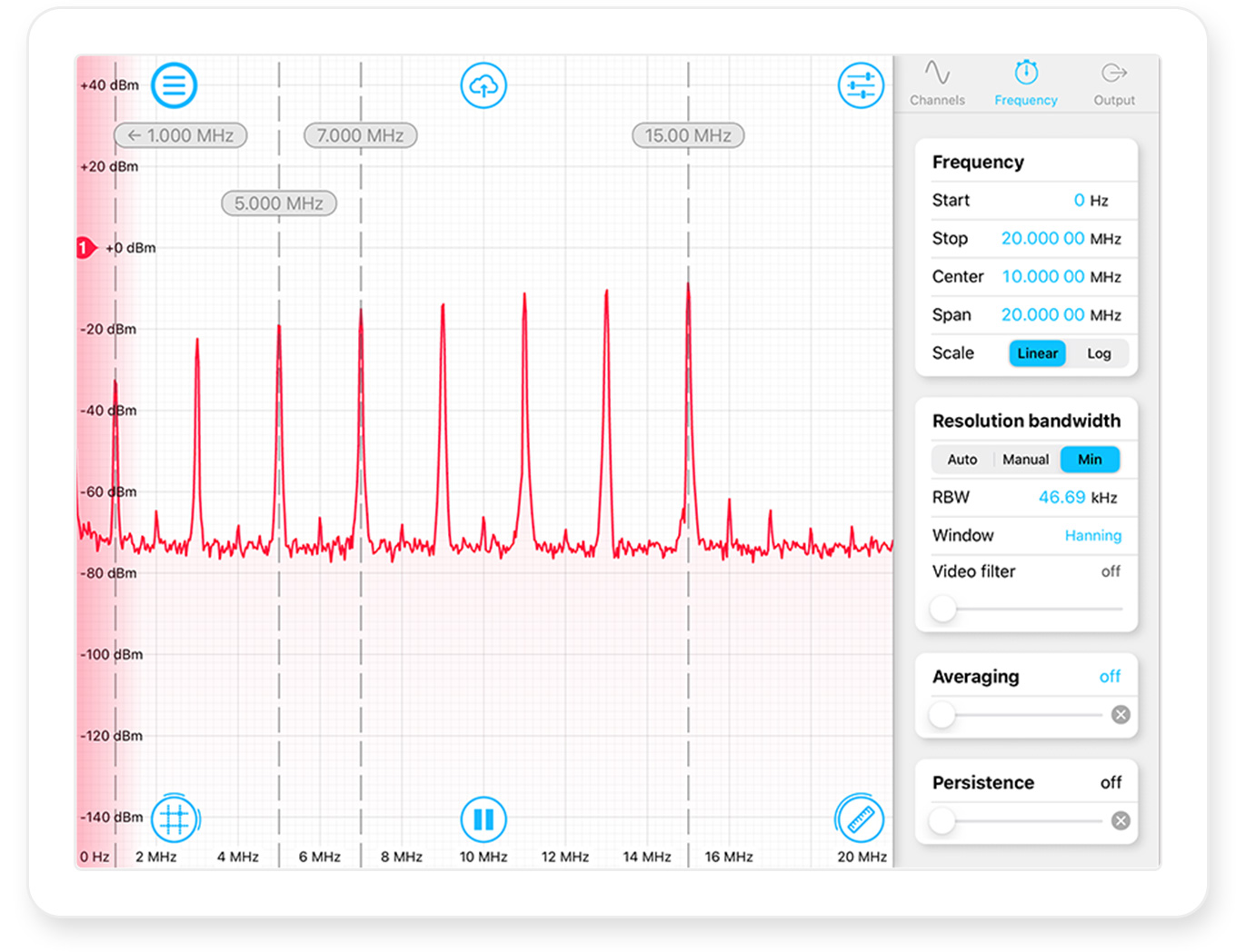Figure 5: “not-square-wave” as seen on Moku:Lab Spectrum Analyzer

## Example #2 : Lissajous figures

For a second example of Moku:Lab’s AWG, we will generate a classic Lissajous figure. Lissajous curves are defined in x and y as:

𝑥=𝐴𝑠𝑖𝑛(𝑎𝑡+𝑑)

𝑦=𝐵𝑠𝑖𝑛(𝑏𝑡)

This family of curves was investigated by Nathaniel Bowditch in 1815, and later in more detail by Jules Antoine Lissajous in 1857.

By integrating some Moku:Lab API function calls into the MATLAB scripts from , we can define some waveforms and see the resulting Lissajous curves in MATLAB. This MATLAB script is

`lissajous_moku.m`

Once running, a GUI window opens in MATLAB and allows the user to configure the variables A, a, d, B, b and t. By clicking on “start”, MATLAB will plot the figures and then a few seconds later, download the waveforms to the connected Moku:Lab’s AWG. Figure 6 shows the MATLAB output and Figure 7 shows the output of Moku:Lab’s AWG as captured on the second Moku:Lab’s Oscilloscope operating in X-Y mode.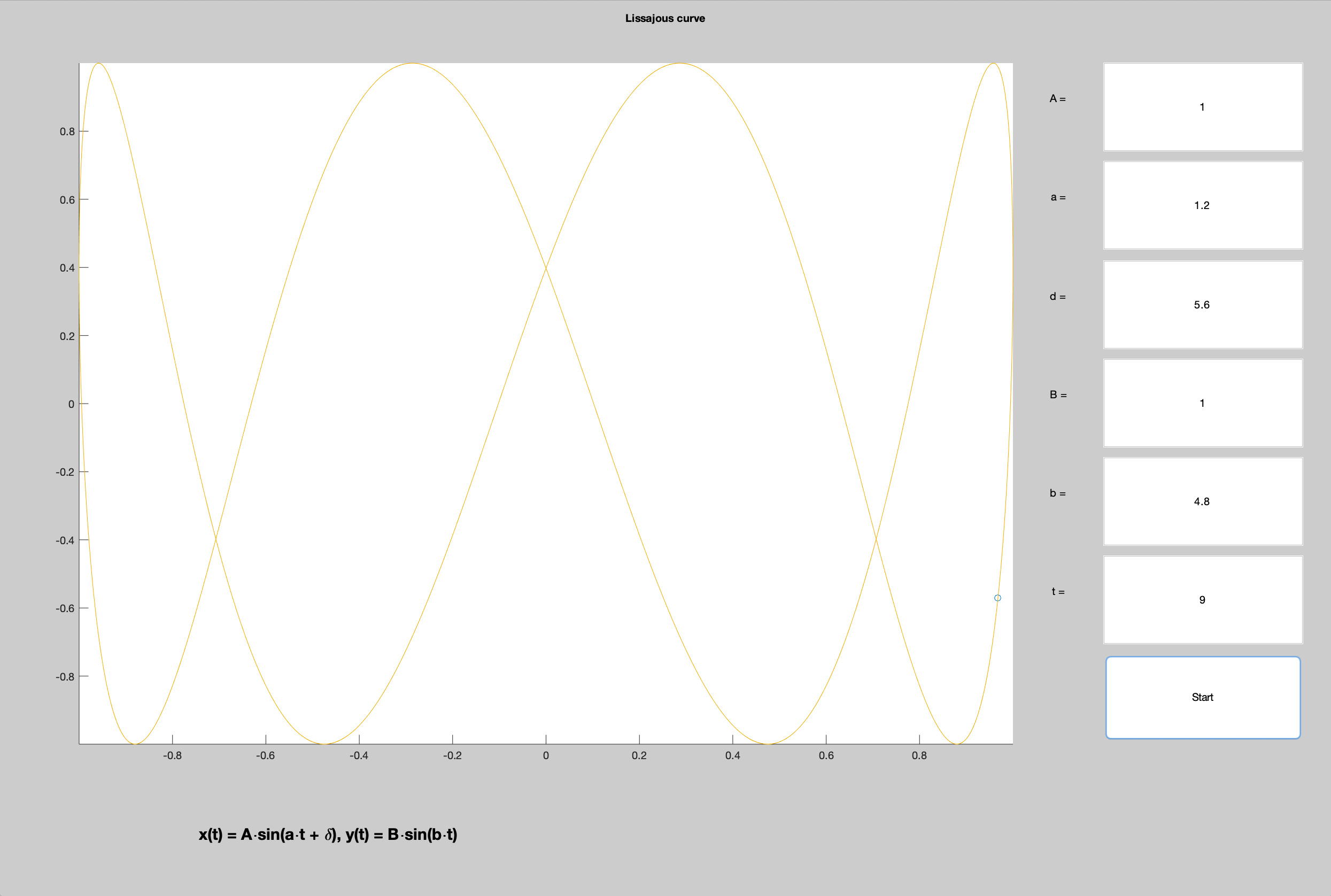Figure 6: MATLAB Lissajous curve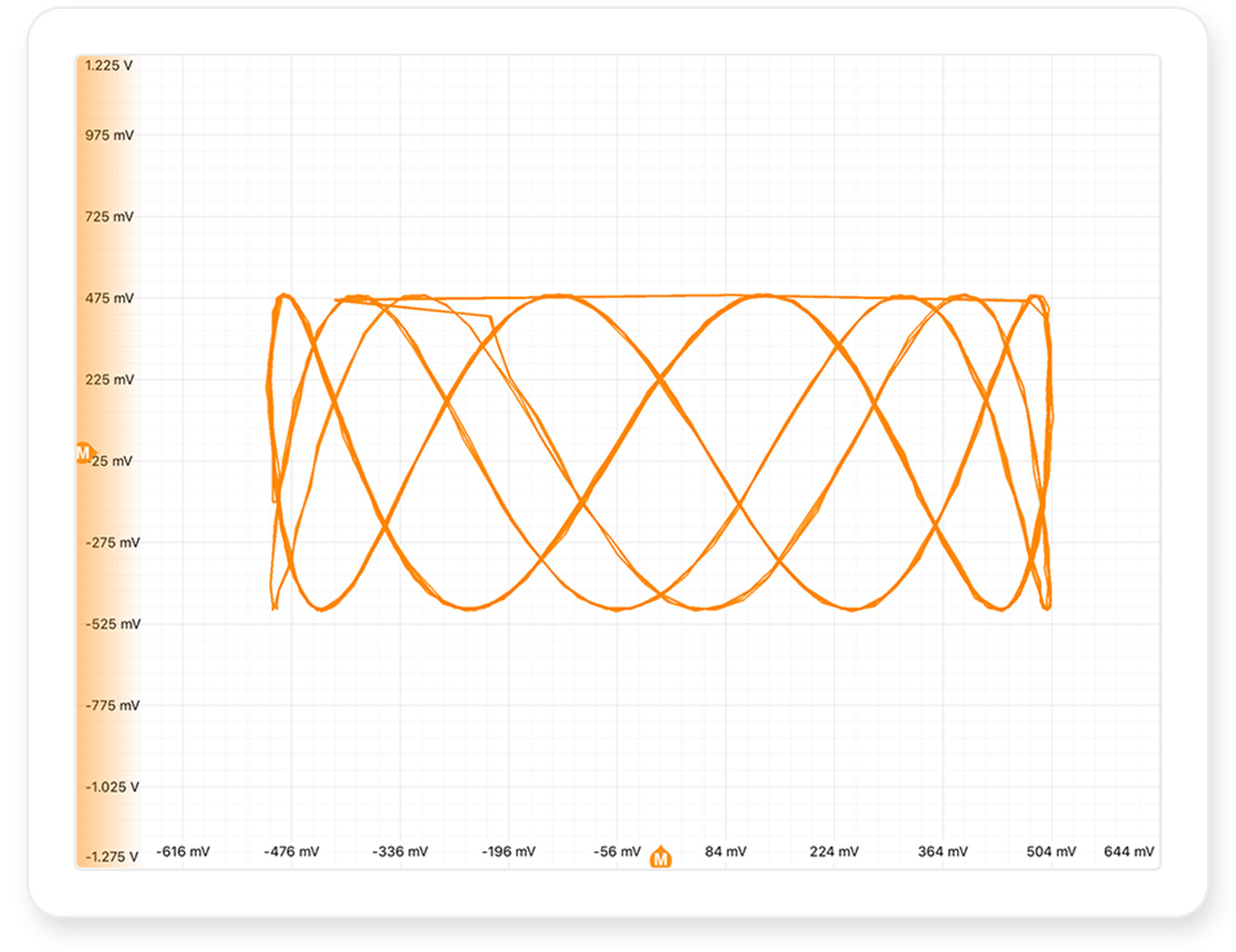Figure 7: Moku:Lab’s AWG showing on Moku:Lab’s Oscilloscope

## Further reading: Dual-channel pattern generation

The application note, “Moku:Lab’s Arbitrary Waveform Generator; dual channel synchronized pattern generation for 2D arbitrary beam steering” ; describes a specific use case of the AWG with MATLAB to define a complex, 2 channel scanning spiral waveform.

## Summary

This application note has shown the flexibility and speed with which you can define a waveform by a mathematical formula in MATLAB. The same MATLAB script seamlessly downloads the waveform to a network-connected Moku:Lab AWG for immediate generation of the described signal.

## References

 Moku:Lab’s AWG User Manual

 Moku:Lab’s Oscilloscope User Manual

 Lissajous curves in MATLAB : https://www.mathworks.com/matlabcentral/fileexchange/28987-lissajous-curve

 Moku:Lab’s Arbitrary Waveform Generator; dual channel synchronized pattern generation for 2D arbitrary beam steering http://download.liquidinstruments.com/documentation/app-note/App%20Note%20-%20MokuLab%20AWG%20Dual%20Channel%20Sync%20Pattern%20Generation.pdf